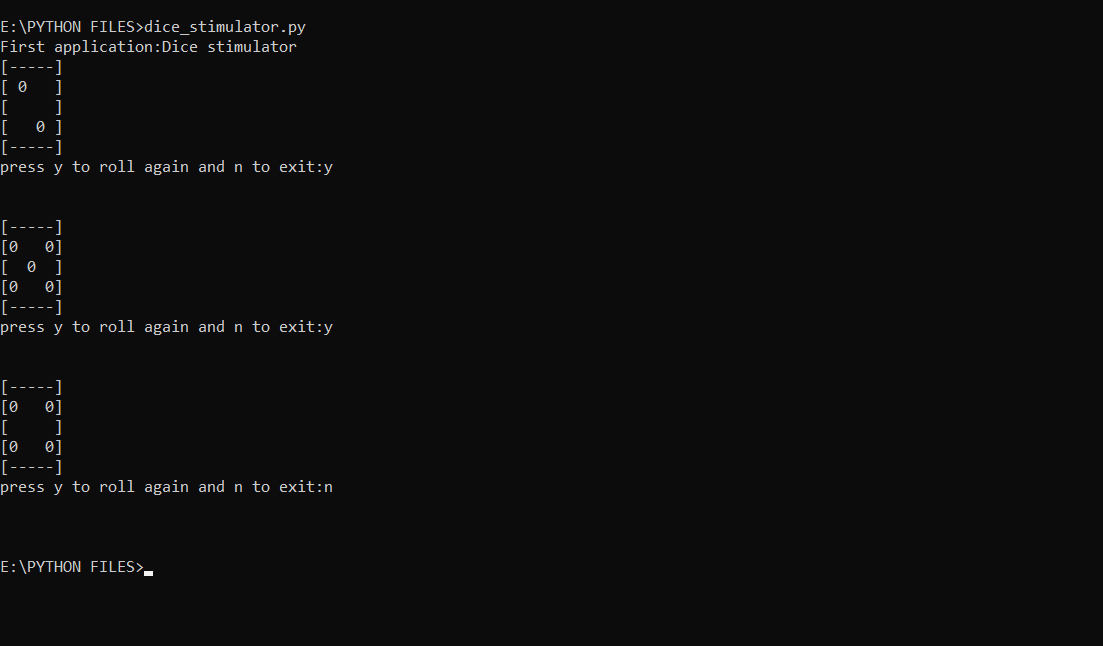Skip to content
Related Articles
Dice Rolling Simulator using Python-random
• Last Updated : 03 Jun, 2020

In this article we will create a classic rolling dice simulator with the help of basic Python knowledge. Here we will be using the random module since we randomize the dice simulator for random outputs.

Function used:

1) random.randint(): This function generates a random number in the given range.

Below is the implementation.

 `import` `random`` ` ` ` `x ``=` `"y"``  ` `while` `x ``=``=` `"y"``:``     ` `    ``# Gnenerates a random number``    ``# between 1 and 6 (including``    ``# both 1 and 6)``    ``no ``=` `random.randint(``1``,``6``)``     ` `    ``if` `no ``=``=` `1``:``        ``print``(``"[-----]"``)``        ``print``(``"[     ]"``)``        ``print``(``"[  0  ]"``)``        ``print``(``"[     ]"``)``        ``print``(``"[-----]"``)``    ``if` `no ``=``=` `2``:``        ``print``(``"[-----]"``)``        ``print``(``"[ 0   ]"``)``        ``print``(``"[     ]"``)``        ``print``(``"[   0 ]"``)``        ``print``(``"[-----]"``)``    ``if` `no ``=``=` `3``:``        ``print``(``"[-----]"``)``        ``print``(``"[     ]"``)``        ``print``(``"[0 0 0]"``)``        ``print``(``"[     ]"``)``        ``print``(``"[-----]"``)``    ``if` `no ``=``=` `4``:``        ``print``(``"[-----]"``)``        ``print``(``"[0   0]"``)``        ``print``(``"[     ]"``)``        ``print``(``"[0   0]"``)``        ``print``(``"[-----]"``)``    ``if` `no ``=``=` `5``:``        ``print``(``"[-----]"``)``        ``print``(``"[0   0]"``)``        ``print``(``"[  0  ]"``)``        ``print``(``"[0   0]"``)``        ``print``(``"[-----]"``)``    ``if` `no ``=``=` `6``:``        ``print``(``"[-----]"``)``        ``print``(``"[0 0 0]"``)``        ``print``(``"[     ]"``)``        ``print``(``"[0 0 0]"``)``        ``print``(``"[-----]"``)``         ` `    ``x``=``input``(``"press y to roll again and n to exit:"``)``    ``print``(``"\n"``)`

Output:Attention geek! Strengthen your foundations with the Python Programming Foundation Course and learn the basics.

To begin with, your interview preparations Enhance your Data Structures concepts with the Python DS Course. And to begin with your Machine Learning Journey, join the Machine Learning – Basic Level Course

My Personal Notes arrow_drop_up